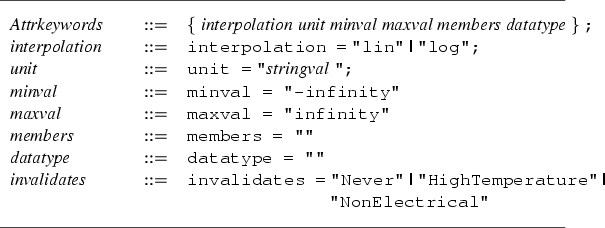# B.3 Definition of the Config File Grammar.

The Config file follows the syntax of the IPD language [24,25]. The purpose of the Config file is to define all kinds of attribute types that may occur in a Wafer. The file is comprised of a tuple that defines the version of the Config file and several sections, one of which -- the Attrkeywords section -- is mandatory and must occur before any other section. The Attrkeywords section defines keywords that are inherited to all other sections in the file. A keyword may be overruled in a sub-section by a simple re-definition of that keyword. The keywords unit and members must be overruled in every sub-section, all other keywords may inherit their default values.

Fig. B.6 depicts the definition of the Attrkeywords section. The basic elements already defined in Fig. B.1 are also used in this grammar definition. A single-line comment is started with two slashes (//) as in the C++ language. Multi-line comments are not supported.A sub-section defines a certain type of attribute (e.g. Concentrations, Forces, Voltage). As already mentioned the members keyword must be overruled. It must contain a list of regular expressions that define all possible names for attributes of that type. The datatype keyword must be overruled to contain the basic data type that is associated with the attribute type (e.g. Scalar, Vector). In the following the Config file currently used in the WAFER-STATE-SERVER is shown. Regular expressions can be used in the members keyword. The Attrkeywords section defines default values for all keywords.

Attrkeywords
{
interpolation = "lin";
unit          = "";
minval        = "-infinity";
maxval        = "infinity";
members       = "";
datatype      = "";
invalidates   = "Never";
};

Concentrations
{
interpolation = "log";
unit          = "1/cm^3";
minval        = 0.;
members       = "Boron_Interstitial, Phosphorus_Interstitial,
Arsenic_Interstitial, Indium_Interstitial,
Antimony_Interstitial, Nitrogen_Interstitial,
Oxygen_Interstitial, Silicon_Interstitial, Boron_Active,
Phosphorus_Active, Arsenic_Active, Indium_Active, Antimony_Active,
Silicon_Vacancy, Oxygen_Vacancy, Nitrogen_Vacancy";
datatype      = "Scalar";
invalidates   = "HighTemperature";
};

InternalForces
{
unit          = "N";
members       = " Stress";
datatype      = "Vector";
invalidates   = "HighTemperature";
};

Epsilon
{
unit          = "As/Vm";
members       = "Epsilon";
datatype      = "Tensor";
};

CurrentDensity
{
unit          = "A/m^2";
members       = "CurrentDensity";
datatype      = "Vector";
invalidates   = "NonElectrical";
};

ElectricField
{
unit          = "V/m";
members       = "ElectricField";
datatype      = "Vector";
invalidates   = "NonElectrical";
};

Voltage
{
unit          = "V";
members       = "Voltage";
datatype      = "Scalar";
invalidates   = "NonElectrical";
};

Charge
{
unit          = "As";
members       = "Charge";
datatype      = "Scalar";
invalidates   = "HighTemperature";
};

Density
{
unit          = "kg/m^3";
members       = "Density";
datatype      = "Scalar";
};

Material
{
unit          = "None";
members       = "MaterialType";
datatype      = "String";
};

Contact
{
unit          = "None";
members       = "Contact";
datatype      = "String";
};

CrystalOrientation
{
unit          = "None";
members       = "CrystalOrientation";
datatype      = "String";
};

Temperature
{
unit          = "K";
members       = "Temperature";
datatype      = "Scalar";
invalidates   = "HighTemperature";
};


2003-03-27